Kundu, A., Redzwan, G., Sahu, J. N., Mukherjee, S., Gupta, B. S., and Hashim, M. A. (2014). "Hexavalent chromium adsorption by a novel activated carbon prepared by microwave activation," BioRes. 9(1), 1498-1518.

#### Abstract

Microwave heating reduces the preparation time and improves the adsorption quality of activated carbon. In this study, activated carbon was prepared by impregnation of palm kernel fiber with phosphoric acid followed by microwave activation. Three different types of activated carbon were prepared, having high surface areas of 872 m2 g-1, 1256 m2 g-1, and 952 m2 g-1 and pore volumes of 0.598 cc g-1, 1.010 cc g-1, and 0.778 cc g-1, respectively. The combined effects of the different process parameters, such as the initial adsorbate concentration, pH, and temperature, on adsorption efficiency were explored with the help of Box-Behnken design for response surface methodology (RSM). The adsorption rate could be expressed by a polynomial equation as the function of the independent variables. The hexavalent chromium adsorption rate was found to be 19.1 mg g-1 at the optimized conditions of the process parameters initial concentration of 60 mg L-1, pH of 3, and operating temperature of 50 ºC. Adsorption of Cr(VI) by the prepared activated carbon was spontaneous and followed second-order kinetics. The adsorption mechanism can be described by the Freundlich Isotherm model. The prepared activated carbon has demonstrated comparable performance to other available activated carbons for the adsorption of Cr(VI).

Hexavalent Chromium Adsorption by a Novel Activated Carbon Prepared by Microwave Activation

Anirban Kundu,a Ghufran Redzwan,a Jaya Narayan Sahu,d Sumona Mukherjee,Bhaskar Sen Gupta,c and Mohd Ali Hashim b,*

Microwave heating reduces the preparation time and improves the adsorption quality of activated carbon. In this study, activated carbon was prepared by impregnation of palm kernel fiber with phosphoric acid followed by microwave activation. Three different types of activated carbon were prepared, having high surface areas of 872 m2 g-1, 1256 m2 g-1, and 952 m2 g-1 and pore volumes of 0.598 cc g-1, 1.010 cc g-1, and 0.778 cc g-1, respectively. The combined effects of the different process parameters, such as the initial adsorbate concentration, pH, and temperature, on adsorption efficiency were explored with the help of Box-Behnken design for response surface methodology (RSM). The adsorption rate could be expressed by a polynomial equation as the function of the independent variables. The hexavalent chromium adsorption rate was found to be 19.1 mg g-1 at the optimized conditions of the process parameters, i.e., initial concentration of 60 mg L-1, pH of 3, and operating temperature of 50 oC. Adsorption of Cr(VI) by the prepared activated carbon was spontaneous and followed second-order kinetics. The adsorption mechanism can be described by the Freundlich Isotherm model. The prepared activated carbon has demonstrated comparable performance to other available activated carbons for the adsorption of Cr(VI).

Keywords: Activated carbon; Palm kernel fiber; RSM; Box-Behnken; Chromium

Contact information: a: Institute of Biological Sciences, University of Malaya, 50603, Kuala Lumpur, Malaysia; b: Department of Chemical Engineering, University of Malaya, 50603, Kuala Lumpur, Malaysia; c: School of Planning, Architecture and Civil Engineering, Queen’s University Belfast, David Keir Building, Belfast, BT9 5AG, UK; d: Department of Petroleum and Chemical Engineering, Faculty of Engineering, Institut Teknologi Brunei, Tungku Gadong, P.O. Box 2909, Brunei Darussalam;

* Corresponding author: alihashim@um.edu.my

INTRODUCTION

Heavy metals, because of their significant potential toxicity and bio-magnification capability, have become one of the most hazardous groups of pollutants in the last century. Efforts to reduce the concentration of heavy metals in liquid effluent have been increasingly stressed for compliance with permissible discharge levels and for fulfilling ethical obligations (Machida et al. 2004). Chromium, like other heavy metals such as lead, zinc, cadmium, and copper, is a toxic metal that is released into the environment through anthropogenic activities. Chromium compounds are used in different industrial processes, such as tanning, electroplating, pigmentation, catalyst for corrosion inhibitors, textile dyeing, steel fabrication, inorganic chemicals, paper and pulp, and wood preservatives (Nguyen et al. 2013). In water, chromium is mainly available in trivalent and hexavalent forms. The hexavalent form of chromium causes cancer and is considered to be more hazardous than its trivalent species (Bello et al. 1999). Prolonged exposure to chromium may cause epigastric pain, nausea and vomiting, severe diarrhea and hemorrhage, and damage to the male reproductive systems of laboratory animals (Agency for Toxic Substances and Disease Registry 2008). Therefore, it is of prime importance to remove chromium from wastewater that is to be discharged to water bodies.

Adsorption with activated carbon is one of the best methods for heavy metal removal; however, according to Demirbas (2009), commercially available activated carbons are normally prepared from non-renewable resources such as coal; therefore, in recent years, interest in the preparation of adsorbents from plant materials has grown. Different adsorbents made from agricultural waste have been used throughout the last decade for removal of Cr(VI) (Ghosh 2009; Karthikeyan et al. 2005; Kumar and Phanikumar 2013; Mohanty et al. 2005; Owlad et al. 2010; Ranjan and Hasan 2010; Sen et al. 2012; Siboni et al. 2011). Palm kernel fiber is a widely available agricultural waste material in Malaysia. In this study, palm kernel fiber was chosen as a precursor material for activated carbon production due to its low cost (Goh et al. 2010).

Activated carbon preparation involves physical or chemical treatment of the precursor material. In both processes, heating the material at high temperature is required, which results in a high consumption of electrical energy (Alslaibi et al. 2013). Because of the thermal gradient from the hot surface of the particle to its core, effective removal of gaseous material is prohibited, which in turn negatively affects the quality of the activated carbon. On the contrary, in the case of microwave heating, heat is produced due to the molecular interaction with the microwave energy. According to one study (Jones et al. 2002) the microwaves penetrate the material and the microwave energy is converted into heat energy, which spreads throughout the bulk of the material and thus can reduce the processing time and improve the overall quality of the adsorbent in terms of high surface area and high pore volume.

In this study, activated carbon was prepared from palm kernel fiber by H3PO4 impregnation followed by microwave activation, which has never been tried before, for Cr(VI) removal. Response surface methodology (RSM) has been used in this work to optimize the number of experiments to study the effect of different operating parameters. A three-factor three-level Box-Behnken (BB) experimental design was used to examine the interaction of the three independent variables (initial adsorbate concentration, initial pH, and temperature) for the removal of Cr(VI) and to optimize the operating conditions. Validity of the suggested model was ascertained by a validation experiment. A kinetic study and an isotherm study were performed at the optimized values of the process variables. A thermodynamic study of the adsorption process was also undertaken.

EXPERIMENTAL

Materials

Palm kernel fibers were collected from a local palm oil mill near Kuala Lumpur, Malaysia, washed to remove dust and dirt, and subsequently dried in an oven at 105 °C until the mass reached a constant value. Phosphoric acid and potassium dichromate of analytical grade were obtained from R&M Chemicals, UK and Fluka Chemicals, respectively. All solutions were prepared in distilled water. A stock solution of chromium of concentration 1000 mg L-1 was prepared with potassium dichromate.The desired concentrations of the Cr(VI) solutions were prepared by diluting the stock solution mentioned above with distilled water.

The precursor material was ground into sizes of 1000 to 2000 µm and washed thoroughly with distilled water. The ground palm kernel fibers were impregnated with phosphoric acid at three different ratios: 1:1, 1:2, and 1:3. Forty grams of the precursor material was mixed with a requisite amount of phosphoric acid for four hours with constant stirring at 120 rpm and at 27 oC. The slurry was then dried in a vacuum oven at 100 oC for 24 h.

The resultant sample loaded with the chemical was placed vertically in a specially designed quartz tube in a microwave oven with a frequency of 2450 MHz (SYNOTHERM corporation, model HAMiLab-C). The microwave power and irradiation time were set at 900 W and 15 min, respectively, based on some preliminary runs. The tube was purged with nitrogen gas at a flow of 0.2 L m-1 for 5 min before microwave treatment to outgas air. This flow rate was maintained during the activation and cooling stages. The product obtained was then washed thoroughly with distilled water until the pH of the washing solution became constant. The activated carbon samples were named MWAC 1, MWAC 2, and MWAC 3 for the ratios 1:1, 1:2, and 1:3, respectively.

Physical and Chemical Characterizations of the Adsorbent

The pore structures of the prepared activated carbons were analyzed using N2 adsorption and scanning electron microscopy (SEM). Nitrogen adsorption/desorption isotherms were used to measure the BET surface area, total pore volume, and density functional theory (DFT) pore size distribution at 77 K using a Quantachrome Autosorb-6B.

A field emission scanning electron microscope (FESEM) (Zeiss Model Auriga) was used to study the surface morphology and pore development. Fourier transform infrared spectroscopy (FTIR, Bruker, IFS66v/S) was used to analyze the surface functional groups of the precursor material and the prepared activated carbons. Spectra were recorded in the range of 400 to 4000 cm−1. Theses analyses were performed following methods mentioned by other researchers (Acharya et al. 2009). The particle sizes of the activated carbon were measured using a Malvern Mastersizer 2000. This instrument uses laser diffraction technology based on the principle that laser scattering occurs when the laser hits a particle and the angle of scattering is directly related to the particle size.

Box–Behnken Experimental Design for Optimization of Adsorption Process by RSM

One of the objectives of this work was to generate an optimum level of output by finding the best settings of the process parameters, which can be achieved with the help of RSM (Pillai et al. 2009). The experimental design, mathematical modeling, and optimization studies were completed using Design Expert 7 software (Stat-Ease, Inc.). The BB design was used to model the experiments since this design require fewer treatment combinations than a central composite design in cases involving 3 or 4 factors. Efficiency of this design is also high and comparable to CCD design (Ferreira et al. 2007). The independent variables used in this study were initial adsorbate concentration, initial pH, and temperature. The three variables were coded A, B, and C, respectively, as shown in Table 1. The ranges of independent variables were designated by -1, 0, and +1, respectively.

Table 1. Summary of the Experimental Design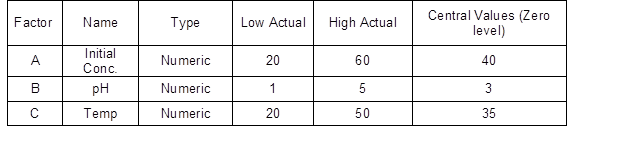Eighteen experiments covering as obtained by the BB design, were performed. The interaction between process variables and the response was determined using analysis of variance (ANOVA) with the help of the same software. The same program determined the quality of fit of the polynomial model, expressed by the coefficient of regression, R2, and its statistical significance was checked by the Fisher F-test (Fisher variation ratio). Model terms were selected or rejected based on the value (probability) with a 95% confidence level. The interactions among the initial adsorbate concentration, initial pH, and temperature were shown in three-dimensional plots. Similar three-dimensional plots were used to elucidate the nature of the interaction between two factors. The first step of RSM requires appropriate approximation with the purpose of finding a true relationship between the experimental parameters and responses. According to Bayraktar (2001), a linear mathematical model, which is insufficient to explain the shape of the response surface, can be upgraded by adding higher-order terms to the preliminary linier equation. Thus, the model can be explained by a quadratic equation defined in Eq. (1) (Adinarayana and Ellaiah 2002; Bayraktar 2001; Mukherjee et al. 2013).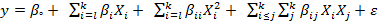(1)

In Eq. (1), y is the response or dependent variable, i and j are linear and quadratic coefficients, β is the regression coefficient, k is the number of factors studied and optimized in the experiment, and ε is the random error.

Experimental Method

Batch adsorption experiments were conducted to eliminate volume correction. Experiments were performed in 250-mL Erlenmeyer flasks containing 100 mL of the metal solution with constant agitation at 120 rpm until equilibrium was reached. Following the adsorption stage, all the samples were filtered through Fioroni 601 filter paper. The final concentration of chromium in the filtrate was measured with ICP-OES (Perkin-Elmer 7000DV). The following equation was applied to calculate the amount of chromium adsorbed,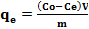(2)

where Co and Ce are the initial and final concentrations of chromium at equilibrium in mg L-1, respectively, m is the mass of adsorbent in grams, and V is the volume of the solution in L.

Kinetic Study and Isotherm Study

In the kinetic experiments, samples were collected at intervals of 10 min for 3 h and the amount of Cr(VI) adsorbed was found using Eq. 2. An attempt was made to fit the obtained adsorption data to Eqs. 3 and 4, which are the standard linearized-integral forms of pseudo-first-order and pseudo-second-order kinetic models, respectively,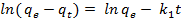(3)

where kl is the Lagergren rate constant of adsorption (min-1). The values of qe and kl were determined from the plot of ln (qe − qt) against t,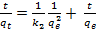(4)

where k2 is the pseudo second-order rate constant of adsorption (g mg-1 min-1). The values of the pseudo-second-order rate constants qe and k2 were calculated from the slopes and the intercepts of the straight portion of the linear plots obtained by plotting t/qt vst.

The isotherm study was conducted with the chromium solution with an initial concentration of 20 to 100 mg L-1. The same amount of adsorbent was added to 100 mL of solution of different concentrations, and the pH was adjusted to 3. To study the adsorption process shake flask experiments were carried out for 24 h at 125 rpm at the fixed temperature, as obtained in the optimization process. To understand the interaction between the metal ion and the adsorbent, a number of models such as Langmuir Isotherm, Freundlich Isotherm, Temkin isotherm, and Dubinin–Radushkevich isotherm models were applied to analyze the experimental data.

The assumption made in the Langmuir isotherm is that the surface with homogeneous binding sites and equivalent sorption energies have no interaction between the adsorbed species. The linearized form of the Langmuir equation can be expressed as follows,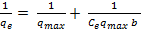(5)

where qmax and represent the maximum adsorption capacity for the adsorbent and the energy constant related to the heat of adsorption, respectively. The values of qmax and were calculated from the slopes and intercepts of the straight line that was obtained by plotting 1/qe vs. 1/C.

The Freundlich Isotherm is an empirical equation based on an exponential distribution of adsorption sites and energies. The linear form of the equation is represented as follows,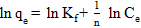(6)

where KF (L g-1) and n are the Freundlich constants related to the adsorption capacity and intensity, respectively. The values of the Freundlich constants were obtained from the slope and intercept of the straight line obtained by plotting ln qe against ln Ce.

The Temkin isotherm model assumes linear decrease in the heat of adsorption with increasing coverage (Dada et al. 2012). The isotherm can be represented by the following equation,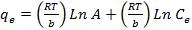(7)

where AbR, and T represent Temkin isotherm equilibrium binding constant (L g-1), Temkin isotherm constant, universal gas constant (8.314J mol-1 K-1), and temperature (298 K), respectively. The constants A and b are calculated from the slope and intercept of the qe against Ln Ce plot.

Dubinin–Radushkevich isotherm model is used to understand the adsorption mechanism with a Gaussian energy distribution onto a heterogeneous surface. The model often successfully relates high solute activities and the intermediate range of concentrations data (Günay et al. 2007). The isotherm can be expressed by the following equation,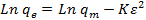(8)

where K (molkJ-2) and 2 are Dubinin–Radushkevich isotherm constants. The saturation adsorption capacity qm and K was calculated from the interception and slope of Ln qe against 2 plot.

RESULTS AND DISCUSSION

Physical and Chemical Characterization of the Adsorbent

Characterization of the prepared activated carbons was conducted using adsorbent pH, average bulk density, BET surface area, SEM, and FTIR analysis. The removal efficiency could be affected by the adsorbent pH. Acidic adsorbent may affect the removal by counter reacting with the material to be removed. In this work, 3 g of the prepared activated carbon was mixed with 30 mL of distilled water and agitated. Then, the value of pH was recorded after 24 h of agitation. The average bulk density of the activated carbon was measured using a water displacement method (Acharya et al. 2009). The values of adsorbent pH, average bulk density, and BET surface areas obtained from the nitrogen adsorption-desorption isotherm are tabulated in Table 2.

Table 2. Physico-Chemical Properties of the Prepared Activated Carbons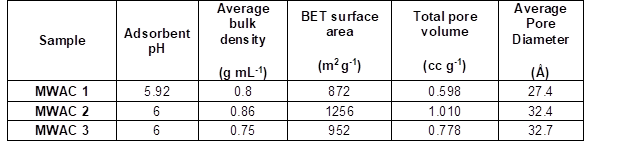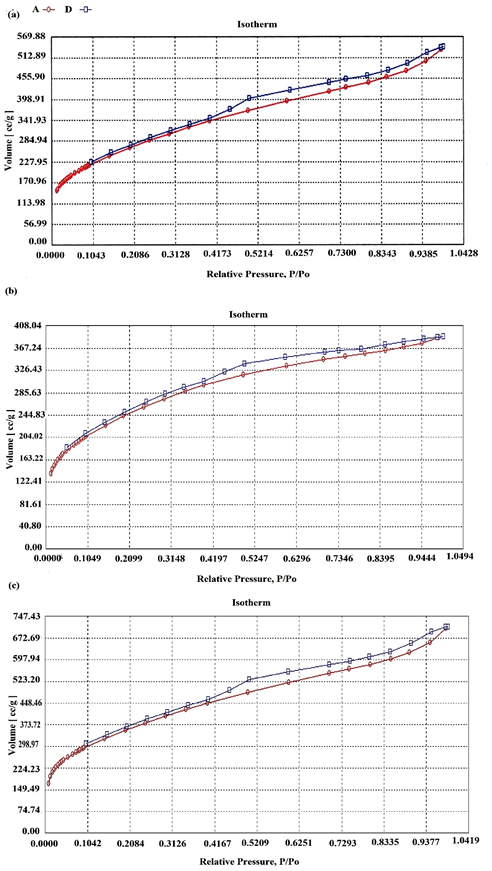Fig. 1. Nitrogen adsorption-desorption isotherms of A) MWAC 1, B) MWAC 2, and C) MWAC 3

MWAC 2 had the highest surface area, of 1256 mg-1. The average pore diameter suggests that the prepared activated carbon is mainly mesoporous. The nitrogen adsorption-desorption isotherms obtained are primarily type II isotherms, as classified by IUPAC (Carmody et al. 2007; Rouquerol et al. 1994), with strong adsorbate-adsorbent interactions representing unrestricted monolayer-multilayer adsorption (Fig. 1). The activated carbons have an average pore width ranging from 4 to 50 Å.

The SEM images describing the surface morphology of the precursor and the three different types of prepared activated carbons are shown in Fig. 2. The precursor had very low porosity on the surface, as can be seen from Fig. 2A; however, development of a highly porous surface due to treatment with phosphoric acid and microwave activation is evident in Fig. 2B, 2C, and 2D.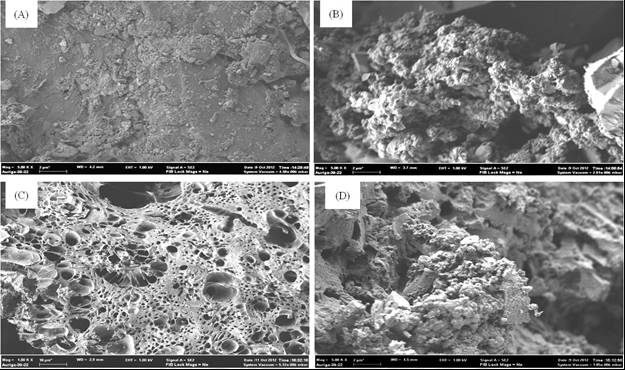Fig. 2. Surface morphology of A) precursor, B) MWAC 1, C) MWAC 2, and D) MWAC 3

The data obtained from FTIR spectroscopy show that peaks at 3452 cm−1 and 2923 cm−1, which were originally obtained for the precursor material, were absent in the data obtained for the three different types of activated carbon. This suggests that the hydrogen part is removed due to carbonization. The data also suggest that the aromatic rings are present in activated carbons as well as in the precursor material because peaks near 1580 cm-1 are visible in both cases.

The results of the characterization study suggested that MWAC 2 had the highest surface area and bulk density among the three prepared activated carbons. Hence, MWAC 2 was chosen for the study of the removal of chromium with the help of response surface methodology.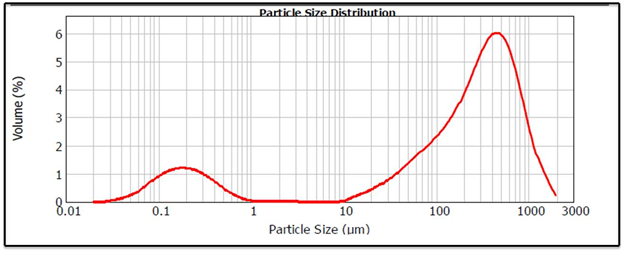Fig. 3. Particle size distribution of the activated carbon MWAC 2

The particle size of the selected activated carbon is distributed between 100 and 1000 µm, as seen in Fig. 3.

Evaluation of the Model and ANOVA of the Chromium (VI) Adsorption Process

An empirical relationship between the response and the independent variables has been expressed by the following quadratic model equation in terms of coded factors:

The results of ANOVA for the response surface reduced quadratic model are presented in Table 3.

Table 3. ANOVA Data for the Second-Order Polynomial Equation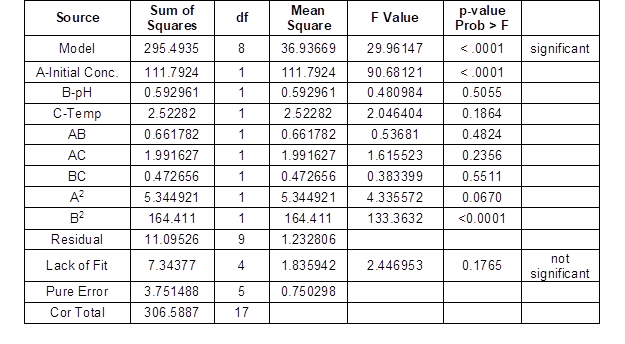ANOVA is an important tool for testing the significance of a model (Sen and Swaminathan 2004). It is a statistical test that compares the means of several groups of data and determines if their means are equal. In a regression analysis, the impact of the independent variables on the dependent variables is determined by ANOVA. As shown in Table 3, ANOVA of the regression model shows that the quadratic model is highly significant for assessing the metal ion removal. This is evident from Fisher’s F-test (F model= 29.96), with a very low probability value of Pmodel > F= 0.0001. This is in conformity with previous analysis (Liu et al. 2004). There is only a 0.01% chance that a model value of this magnitude could occur due to noise. In the graph of the predicted values versus actual data points, the 45-degree line should evenly split the data set. In this case, one can see from Fig. 4A that the points are evenly distributed around the 45-degree line, which suggests that the model can predict the response with values very close to the experimentally obtained values.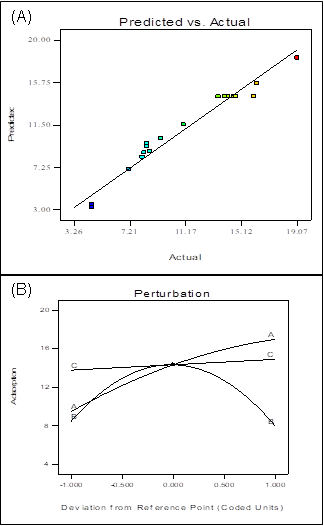Fig. 4. A) Predicted vs. actual plot and B) perturbation plot, showing the effect of all variable parameters on adsorption

The accuracy of prediction of a response value by a model can be measured by the predicted R2. For the model to be sufficient, a difference of less than 0.20 should exist between the observed and the predicted data. If the predicted and adjusted R2 values are not within approximately 0.20, then there is an error in the model or the data. In the case of adsorption, the predicted R2 value is 0.7504, which is within reasonable agreement with the adjusted R2 value of 0.9316.

A signal-to-noise ratio indicates the preciseness of the model. The signal-to-noise ratio is an assessment of the range of the predicted response relative to the associated error. The error expressed as a percentage of the mean indicates the coefficient of variation for this model. According to Aghamohammadi et al. (2007), the desired value should be 4 or more. The ratio of 19.094, in this case, indicates an adequate signal.

The perturbation plot helps to understand the effect of the independent factors at a particular point of the design space. A perturbation plot illustrates the change in the response while each factor moves from a chosen reference point, generally the midpoint, keeping all other factors constant at their reference value (Sen Gupta and Ako 2005). The plot of the response (adsorption) is carried out against the deviation from the reference point by changing only one factor over its entire range while holding all other factors constant, as shown in Fig. 4B. The nature of the plots of all three independent variables suggests that the adsorption is sensitive to the process variables, especially initial concentration and pH.

Combined Effect of Initial Concentration and pH

The three-dimensional plot of interaction of initial Cr(VI) concentration and initial operating pH of the solution keeping the temperature (35 oC) as the actual factor is illustrated in Fig. 5A. The adsorption of Cr(VI) increased as the initial concentration increased for the entire range of pH values used in this work. The 3D plot shows that the adsorption was maximum around a pH of 3. This may be because at pH 3 the negatively charged species HCrO4 and Cr2O72− were prevalent in the solution and were attracted by the protonated surface of the activated carbon. At a lower pH, the neutral species H2CrO4prevails (Gherasim et al. 2011); therefore, the attraction by the protonated surface is lower than the attraction at pH 3. The adsorption capacities at pH 1 and 5 were 11.54 mg gm-1 and 10.18 mg gm-1,respectively, at an initial Cr(VI) concentration of 60 mg L-1. The adsorption capacity of the adsorbent increased to 16.98 mg gm-1 at pH 3 with the same concentration of Cr(VI). Adsorption was also reduced at a higher pH due to electrostatic repulsion between the OH ions and negatively charged HCrO4 and Cr2O72− ions, as reported by Kiran et al. (2007) and Prasad and Abdullah (2010). Chromium exists in different oxidation states and the stability of these forms depends upon the pH of the system. Aqueous chemistry of Cr(VI) suggests that Cr(VI) can be reduced into Cr(III) at low pH value according to the following chemical reaction: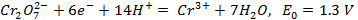(9)

In order to determine the amount of Cr(III) formed during the adsorption process the following test was performed: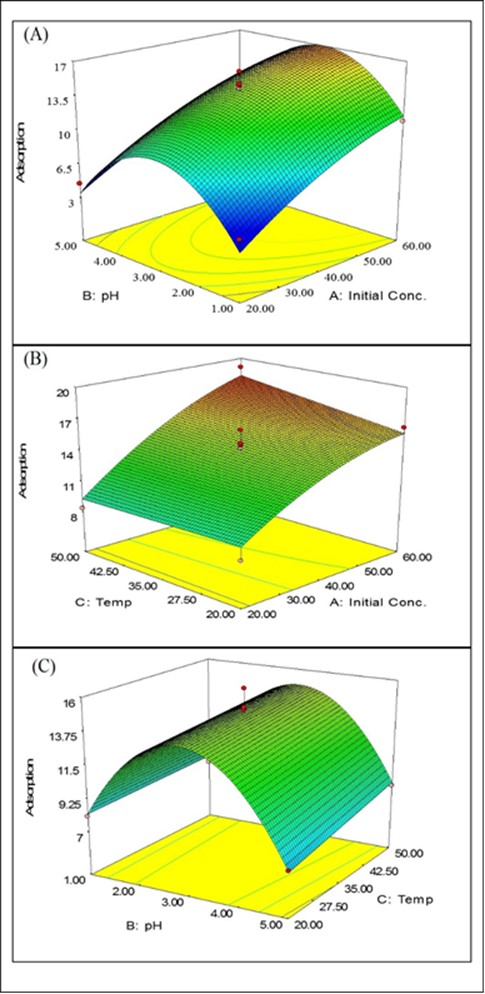Fig. 5. A) Combined effect of initial concentration and pH for chromium adsorption; B) combined effect of initial concentration and temperature; and C) combined effect of pH and temperature

To 100 mL solution of K2Cr2O7 of concentration 20 mg/L, taken in a conical flask, 0.1 g of MWAC2 was added. It was then shaken for 24 h. It was then filtered and divided into two parts. The first part was tested directly by ICP for Cr(VI) concentration. Let the concentration be [Cr]a. Ten milliliters of the sample from the other part was taken in a test tube and heated and excess NaOH and H2O2 was added. This solution was filtered and tested in ICP for Cr(VI) concentration. Let the concentration be [Cr]b. The Cr(III) concentration can be found out by the difference of [Cr]a and [Cr]b. The chemistry is as follows: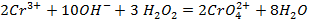(10)

The concentrations of the samples [Cr]and [Cr]b were found to be 2.58 mgL-1 and 3.38 mgL-1, respectively. Hence, the equilibrium concentration values ascertain that small amount of Cr(III) was formed during the adsorption process and remained in the liquid. Similar results were observed by (Gupta et al. 2010).

Combined Effect of Initial Concentration and Temperature

The combined effect of temperature and initial Cr(VI) concentration can be observed from the three-dimensional plots shown in Fig. 5B, where pH is the dominant factor. At a lower concentration of chromium, temperature had little effect on adsorption; however, at a higher initial concentration, the temperature change was significant for adsorption of Cr(VI). The adsorption capacity increased to 19.1 mg gm-1 from 8.39 mg gm-1 as the temperature increased from 20 oC to 50 oC, and the initial concentration increased from 20 mg L-1 to 60 mg L-1 of Cr(VI). The increase in adsorption capacity with increasing temperature may be attributed to the increased adsorbate transfer within the pores of the adsorbent. It may also happen due to an increase in the number of adsorption sites generated at the surface at the increased temperature (Acharya et al. 2009).

Combined Effect of pH and Temperature

As can be seen in Fig. 5C, the adsorption capacity was maximized at pH 3. The adsorption capacity increased with temperature. The amount of adsorbed Cr(VI) increased from 15.71 mg gm-1 to 18.25 mg gm-1 as the temperature increased to 50 oC from 20 oC at pH 3 and an initial concentration of 60 mg L-1 of Cr(VI).

The optimization of the process parameters such as initial Cr(VI) concentration, initial operating pH, and operating temperature for maximum Cr(VI) adsorption was accomplished by a multiple response method called desirability function. The criteria for the independent and dependent variables (adsorption in mg gm-1) are set as “in range” and “maximum” respectively. The desirability values were found to be 0.943232 and 1 for combination and individual variables, respectively.

As suggested by Design Expert the optimum removal of Cr(VI) should be 18.25 mg gm-1 when the independent variables are set at an initial concentration of Cr(VI) of 60 mg L-1, pH of 3, and temperature of 50 oC at the maximum desirability value. Confirmatory experiments were performed at the optimum values of the process parameters to validate the model. The adsorption was found to be 19.1 mg gm-1 in the validation experiment. A summary of the different plant-based materials used as adsorbents and the range of process conditions for Cr(VI) adsorption are given in Table 4, which shows that the prepared activated carbon in this study has comparable adsorption capacity with other plant-based adsorbents used in different studies.

Table 4. A Summary of the Different Plant-Based Materials used as Adsorbents and the Range of Process Conditions for Cr(VI) Adsorption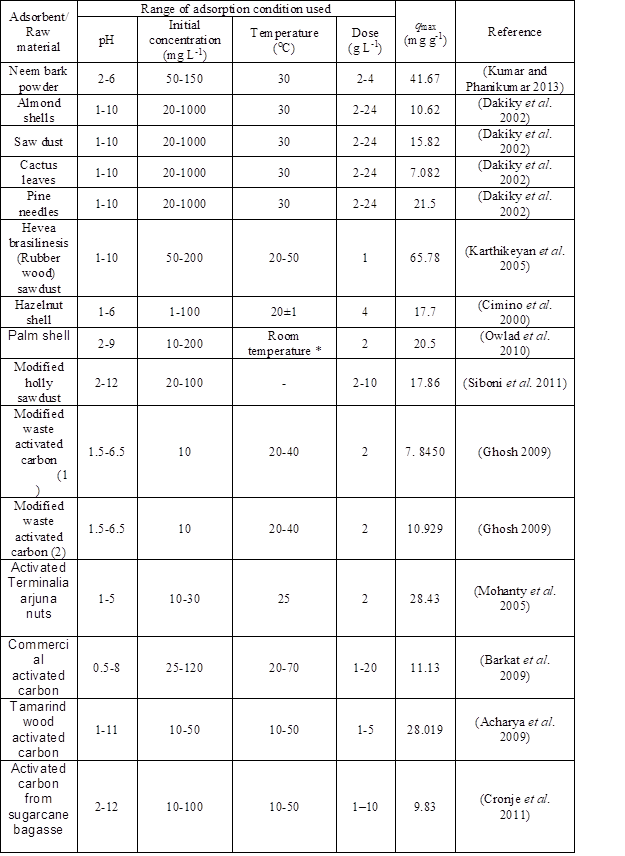* (exact temperature not mentioned)

Kinetic Study

The data obtained from the kinetic experiments were validated with a pseudo-first-order kinetic equation, popularly known as the Lagergren equation, as well as a pseudo-second-order kinetic equation. The results obtained after regression analysis of the data collected for the pseudo-first-order and pseudo-second-order kinetics are shown in Fig. 6A and 6B, respectively. Applicability of a particular type of rate equation is selected based on the value of regression coefficient R2. The value of regression coefficients and rate constants are tabulated in Table 5. The R2 value of the pseudo-second-order equation (0.9931) suggests that this could describe the adsorption of chromium better than the pseudo-first-order equation, which has an R2 value of 0.8046. Therefore it can be said that the rate limiting step in the adsorption is mainly chemisorption which involves valence forces, occurred due to sharing or exchange of electrons between adsorbent and adsorbate (Kushwaha et al. 2008).

Table 5. Values of Kinetic Model Parameters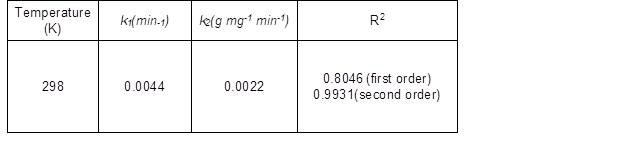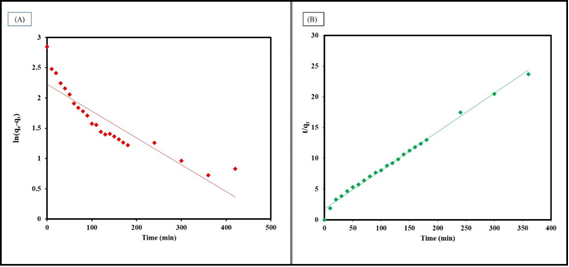Fig. 6. A) Pseudo-first-order equation and B) pseudo-second-order equation

In the equilibrium state, the distribution of molecules between the adsorbent and the adsorbate is generally governed by the adsorption isotherm. The two most extensively used models are the Langmuir and Freundlich isotherms. The Langmuir Isotherm model considers a monolayer adsorption onto a surface composed of a finite number of adsorption sites with no trans-migration of adsorbate in the plane of the surface. In contrast, heterogeneous surface energies are considered in the Freundlich Isotherm model. Here, the energy term in the Langmuir equation varies as a function of the surface coverage (Weber 1972). Figure 7 represents the plots of all the four isotherms that have been tested for the obtained data.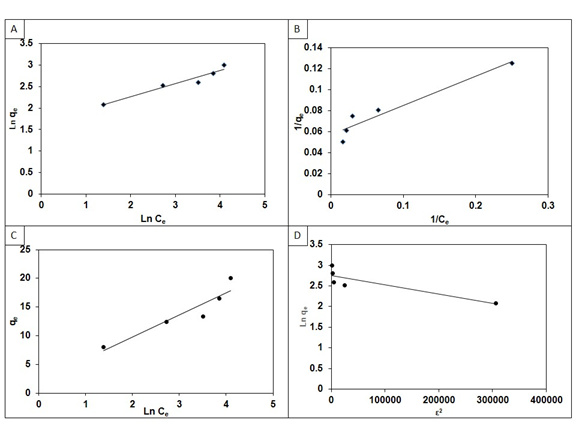Fig. 7. A) Freundlich Isotherm, B) Langmuir Isotherm, C) Temkin Isotherm, and D) Dubinin–Radushkevich Isotherm plots for the adsorption of Cr(VI) by MWAC2

The isotherm parameters of the two models are given in Table 6.

Table 6. Values of Isotherm Model Parameters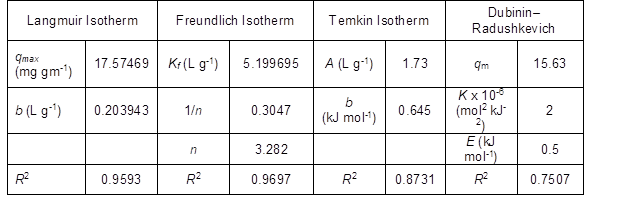From the R2 values, it is suggested that the adsorption process follows the Freundlich Isotherm model. The value of n is 3.282, which suggests favorable conditions for the adsorption as 1<n<10 (Kumar and Phanikumar 2013). The dimensionless parameter RL was calculated from the following equation.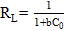(7)

The value of RL also suggests the favorability of adsorption. It may be noted that RL > 1 suggests an unfavorable process, whereas 0<RL<1 suggests a favorable process. The RL values for initial concentrations of 20 mg L-1, 40 mg L-1, 60 mg L-1, 80 mg L-1, and 100 mg L-1 were found to be 0.1969, 0.1092, 0.0755, 0.0578, and 0.0467, respectively, which suggests that the adsorption process is favorable. The Rvalues of Temkin and Dubinin–Radushkevich isotherm models were less than other two; hence it could be concluded that the data did not fit well with these isotherm models.

Thermodynamic Study

Thermodynamic parameters such as change in free energy (G0), enthalpy change (H0), and entropy change (S0) help to characterize the nature of the adsorption to its full extent.

The following equations are employed to measure the change in free energy (∆G°) for an adsorption process,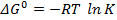(8)